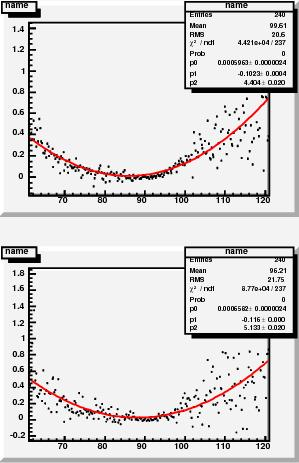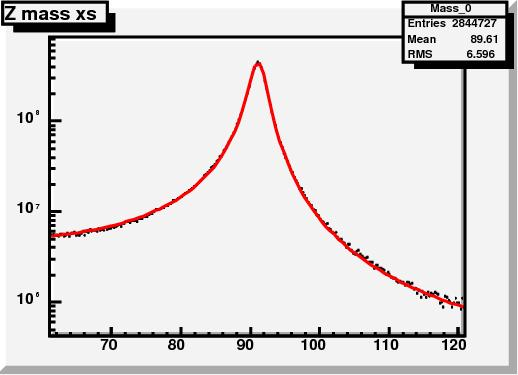## Guess the function: resultsJanuary 21, 2009

Posted by dorigo in physics, science.
Tags: , , , , , ,

Thanks to the many offers for help received a few days ago, when I asked for hints on possible functional forms to interpolate a histogram I was finding hard to fit, I have successfully solved the problem, and can now release the results of my study.

The issue is the following one: at the LHC, Z bosons are produced by electroweak interactions, through quark-antiquark annihilation. The colliding quarks have a variable energy, determined by probability density functions (PDF) which determine how much of the proton’s energy they carry; and the Z boson has a resonance shape which has a sizable width: 2.5 GeV, for a 91 GeV mass. The varying energy of the center of mass, determined by the random value of quark energies due to the PDF, “samples” the resonance curve, creating a distortion in the mass distribution of the produced Z bosons.

The above is not the end of the story, but just the beginning: in fact, there are electromagnetic corrections (QED) due to the radiation of photons, both “internally” and by the two muons into which the Z decays (I am focusing on that final state of Z production: a pair of high-momentum muons from$Z \to \mu^+ \mu^-$). Also, electromagnetic interactions cause a interference with Z production, because a virtual photon may produce the same final state (two muons) by means of the so-called “Drell-Yan” process. All these effects can only be accounted for by detailed Monte Carlo simulations.

Now, let us treat all of that as a black box: we only care to describe the mass distribution of muon pairs from Z production at the LHC, and we have a pretty good simulation program, Horace (developed by four physicists in Pavia University: C.M. Carloni Calame, G. Montagna, O. Nicrosini and A. Vicini), which handles the effects discussed above. My problem is to describe with a simple function the produced Z boson lineshape (the mass distribution) in different bins of Z rapidity. Rapidity is a quantity connected to the momentum of the particle along the beam direction: since the colliding quarks have variable energies, the Z may have a high boost along that direction. And crucially, depending on Z rapidity, the lineshape varies.

In the post I published here a few days ago I presented the residual of lineshape fits which used the original resonance form, neglecting all PDF and QED effects. By fitting those residuals with a proper parametrized function, I was trying to arrive at a better parametrization of the full lineshape.

After many attempts, I can now release the results. The template for residuals is shown below, interpolated with the function I obtained from an advice by Lubos Motl:After multiplying that function by the original Breit-Wigner resonance function, I could fit the 24 lineshapes extracted from a binning in rapidity. This produced additional residuals, which are of course much smaller than the first-order ones above, and have a sort of parabolic shape this time. A couple of them are shown on the right.

I then interpolated those residuals with parabolas, and extracted their fit parameters. Then, I could parametrize the parameters, as the graph below shows: the three degrees of freedom have roughly linear variations with Z rapidity. The graphs show the five parameter dependences on Z rapidity (left column) for lineshapes extracted with the CTEQ set of parton PDF; for MRST set (center column); and the ratio of the two parametrization (right column), which is not too different from 1.0.Finally, the 24 fits which use the$f(m,y)$ shape, with now all of the rapidity-dependent parameters fixed, are shown below (the graph shows only one fit, click to enlarge and see all of them together).The function used is detailed in the slide below:I am rather satisfied by the result, because the residuals of these final fits are really small, as shown on the right: they are certainly smaller than the uncertainties due to PDF and QED effects. The$f(m,y)$ function above will now be used to derive a parametrization of the probability that we observe a dimuon pair with a given mass$m$ at a rapidity$y$, as a function of the momentum scale in the tracker and the muon momentum resolution.